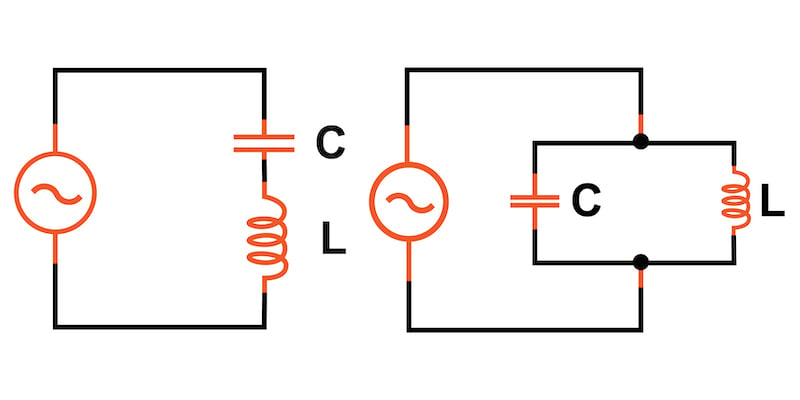# LC Resonant Frequency Calculator

## A circuit with an inductor (L) and capacitor (C) connected in parallel or series will have a resonant frequency at which their impedances are equal. Given two of the three values—inductance, capacitance, or resonant frequency—this tool will calculate the missing third variable.

### Calculating the Resonant Frequency of an LC Circuit

When an inductor and capacitor are connected in series or parallel, they will exhibit resonance when the absolute value of their reactances is equal in magnitude.##### Series and parallel LC circuits

The reactances or the inductor and capacitor are given by:

$$X_L = 2 \pi f L$$

$$X_C = \frac{1}{(2 \pi f C)}$$

Where:

• XL = inductor reactance
• XC = capacitor reactance
• L = inductance in H
• C = capacitance in F
• f = resonant frequency in Hz

Setting XL = XC and solving for the resonant frequency results in the following equation:

$$f = \frac{1}{(2\pi\sqrt{LC})}$$

Alternatively, if we have a target resonant frequency and know either the inductance or capacitance, we can solve for the other required component value:

$$L = (\frac{1}{C}) · (\frac{1}{(2 \pi f)})^2$$

and

$$C = (\frac{1}{L}) · (\frac{1}{(2 \pi f)})^2$$

### Applications

The resonant effect of LC circuits has many important applications in analog and RF signal processing including use in notch filters, bandpass filters, and oscillators.

Textbook—Simple Series Resonance

Textbook—Resonance in Series-Parallel Circuits